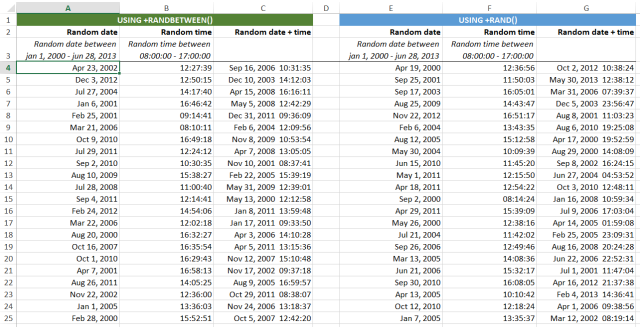## Insert random dates and times in Excel

We just received the following question:
"Would it be possible to add random date and times?"

Yes, this can (already) be done with just Excel.
We might add a tool for it in ASAP Utilities that will ask you for the input in the future, but it isn't hard to do yourself with the combination of a few Excel formulas.

These are a few examples of how you can generate random dates and times in Excel within a specified period.
(Please note: After you insert the formula you need to set the cell's number-formatting to date/time, otherwise you'll just see numbers.)
Insert a random date between Jan 1, 2000 and June 28, 2013:

`=RANDBETWEEN(DATE(2000,1,1),DATE(2013,6,28))`

Insert a random time between 8 AM and 5 PM:

`=RANDBETWEEN(TIME(8,0,0)*10000,TIME(17,0,0)*10000)/10000`

Insert a random time between 08:00 and 17:00:

`=TIME(8;0;0)+RAND()*(TIME(17;0;0)-TIME(8;0;0))`

Insert a random date+time between Jan 1, 2000 and June 28, 2013 with a time between 8:00 and 17:00:

`=RANDBETWEEN(DATE(2000,1,1),DATE(2013,6,28))+RANDBETWEEN(TIME(8,0,0)*10000,TIME(17,0,0)*10000)/10000`

Insert a random date+time between Jan 1, 2000 and June 28, 2013:

`=DATE(2000,1,1)+(RAND()*DATE(2013,6,28)-(DATE(2000,1,1)))`

(Some example uses the =RANDBETWEEN() Excel function. If you have Excel 2003 or earlier then you have to load/activate the Analysis ToolPak add-in which comes with Excel and is from Microsoft).

If you have a non-English version of Excel or use a different separator than the comma, then you can use the following tool to easily insert these example formulas in your Excel workbook.
The formula will then automatically be converted to your local Excel language and settings:
ASAP Utilities » Formulas » Insert "international" formula...

You can download an example workbook that demonstrates how to insert random dates and times:
Example - Random dates and times.xls (40kb)## Resources

The following two articles explain how you can insert random dates:

Dummy Data – How to use the Random Functions
http://chandoo.org/wp/2011/05/04/dummy-data-random-functions/

Generate random dates within a specific date range
http://www.techrepublic.com/blog/msoffice/generate-random-dates-within-a-specific-date-range/8153

#### Bastien

Website: https://www.asap-utilities.com